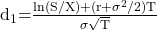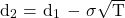Essential Concept 80: The BSM Model | IFT World
101 Concepts for the Level I Exam

# Essential Concept 80: The BSM ModelThe inputs to the BSM model are:

• Price of underlying stock, S
• Continuously compounded risk-free rate, r
• Time to maturity in years, T
• Strike price, X
• Volatility of the underlying in annual percentage terms, σ

The BSM model for non-dividend paying stock is:

c = SN(d1) – e–rTXN(d2)

p = e–rTXN(–d2) – SN(–d1)

where:Using the above inputs, the BSM model can be used to predict:

• Call option price, c
• Put option price, p

Instructor’s Note: